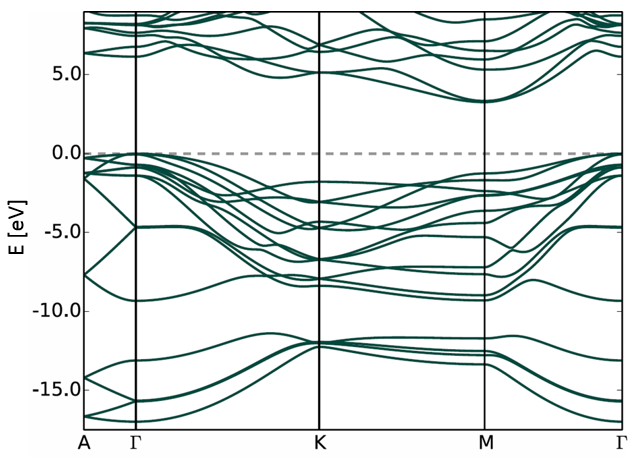513.160 Microelectronics and Micromechanics

## SiC 4H

Silicon carbide 4H.

### Structural properties

Crystal structure: SiC 4H
Bravais lattice: hexagonal
Space group: 186 (P 63 m c),
Point group: 6mm (C6v) one 6-fold rotation, six mirror planes, no inversion

The conventional unit cell is:

$\begin{equation} a = 0.3073\, \text{nm}, \, b = 0.3073 \,\text{nm}, \, c = 1.0053 \,\text{nm}, \, \alpha = 90^{\circ} , \,\beta = 90^{\circ} ,\,\gamma = 120^{\circ} . \end{equation}$

Space group: 186 (P 63 m c)
12 symmetry operations:

1	x,y,z	identity
2	-x,-x+y,z+1/2	c-glide plane|translation: 0 0 1/2
3	x-y,-y,z+1/2	c-glide plane|translation: 0 0 1/2
4	y,x,z+1/2	c-glide plane|translation: 0 0 1/2
5	x-y,x,z+1/2	6-fold screw axis|translation: 0 0 1/2
6	y,-x+y,z+1/2	6-fold screw axis|translation: 0 0 1/2
7	-x,-y,z+1/2	2-fold screw axis|translation: 0 0 1/2
8	x,x-y,z	mirror plane
9	-x+y,y,z	mirror plane
10	-y,-x,z	mirror plane
11	-x+y,-x,z	C3 axis
12	-y,x-y,z	C3 axis



### Electrical properties

SiC 4H is an indirect bandgap semiconductor with a bandgap of 3.3 eV.Calculated using FHI-aims by Lydia Nemec.